Question

IDY in < oo and lim - Yn < 0o. Prove that lim,+ 1. Let In...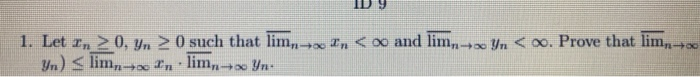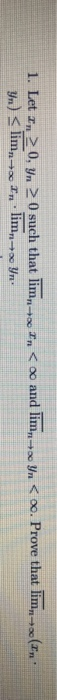IDY in < oo and lim - Yn < 0o. Prove that lim,+ 1. Let In > 0. Yn > 0 such that lim,- Yn) < lim,-- In lim,+ Yn:
i tn < oo and lim yn < . Prove that lim. In 1. Let In 20, yn 0 such that lim Yn) < limn+In lim + Yr

Sol: Here, given that,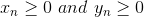.

This implies that the sequences {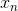} and {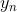} are bounded.

Also, given that,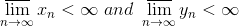When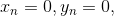we get,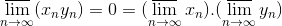Which follows the inequality.

Now, we will proof the inequality for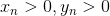Let,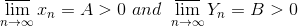Also, we know that, a real number A is the limit superior of a bounded sequence {} if and only if for each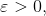there exists a positive integer m such that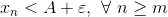So, here, for somethere exists positive integers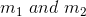such that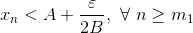and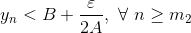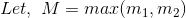.

Therefore, for all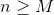, we get,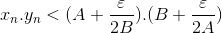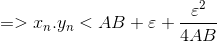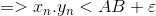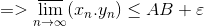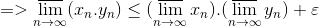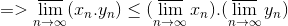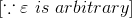We were unable to transcribe this image

We were unable to transcribe this image

We were unable to transcribe this image

We were unable to transcribe this image

In = 0, yn = 0.

We were unable to transcribe this image

We were unable to transcribe this image

We were unable to transcribe this image

We were unable to transcribe this image

We were unable to transcribe this image

We were unable to transcribe this image

We were unable to transcribe this image

We were unable to transcribe this image

We were unable to transcribe this image

We were unable to transcribe this image

We were unable to transcribe this image

We were unable to transcribe this image

We were unable to transcribe this image

We were unable to transcribe this image

We were unable to transcribe this image

We were unable to transcribe this image

We were unable to transcribe this image

We were unable to transcribe this image

We were unable to transcribe this image

Earn Coins

Coins can be redeemed for fabulous gifts.

Similar Homework Help Questions
• (b) Show that if lim sn oo and lim tn > -oo, then lim(sn + tn)...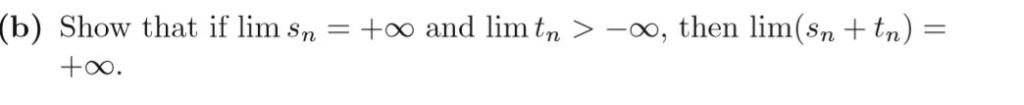(b) Show that if lim sn oo and lim tn > -oo, then lim(sn + tn) 1 +oo.

• {x_n} and {y_n} are sequences of positive real numbers AC fn→oo > O, prove tha m...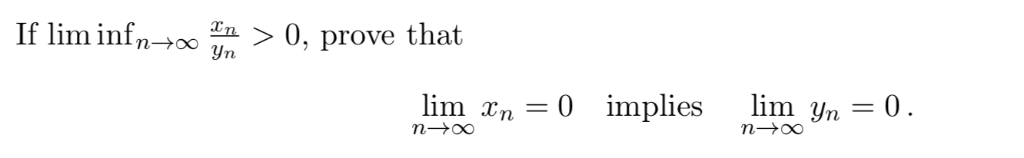{x_n} and {y_n} are sequences of positive real numbers AC fn→oo > O, prove tha m in yn lim xn 0 implies lim yn_0

• , then n lim Let Ά be a square matrix. Prove that if ρ(A)<1 Use the...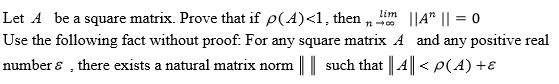, then n lim Let Ά be a square matrix. Prove that if ρ(A)<1 Use the following fact without proof. For any square matrix A and any positive real number ε , there exists a natural matrix norm I l such that l-4 ll < ρ (d) +ε IIA" 11-0

• Let (In), and (yn).m-1 be sequences such that Pr – yn| < 1/n for all n....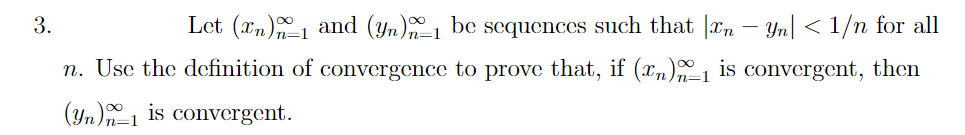Let (In), and (yn).m-1 be sequences such that Pr – yn| < 1/n for all n. Use the definition of convergence to prove that, if (2n)_1 is convergent, then (Yn)-1 is convergent.

• Please prove this, thanks! 2. Let {xn n21 be a sequence in R such that all...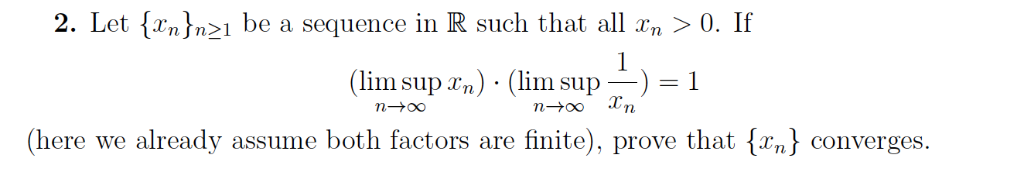Please prove this, thanks! 2. Let {xn n21 be a sequence in R such that all n > 0. If ( lim supra) . (lim supー) = 1 Tn (here we already assume both factors are finite), prove that converges.

• Let U ? Rmxn. Prove that if UTI-In, then n < m.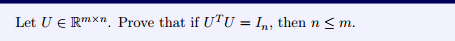Let U ? Rmxn. Prove that if UTI-In, then n < m.

• 6. Let si = 4 and sn +1 (sn +-) for n > 0. Prove lim...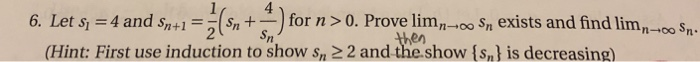6. Let si = 4 and sn +1 (sn +-) for n > 0. Prove lim n→oo sn exists and find limn-oo Sn. (Hint: First use induction to show sn 2 2 and the.show (sn) is decreasing)

• Compute limn >oo A" for 1 1/2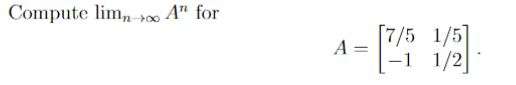Compute limn >oo A" for 1 1/2

• Let 0 <10 <yo and In+1 = Vonyn Yn+1 = = (en + yn). Prove that...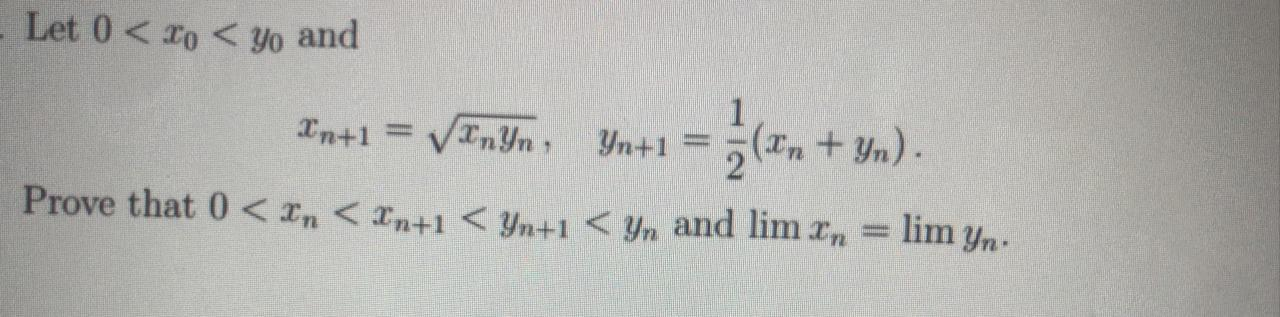Let 0 <10 <yo and In+1 = Vonyn Yn+1 = = (en + yn). Prove that 0 < xn < In+1 < 4n+1 < yn and lim x, = lim yn. n1

• Let ne Nj. Prove that n < 2(6(n)).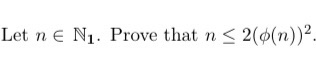Let ne Nj. Prove that n < 2(6(n)).